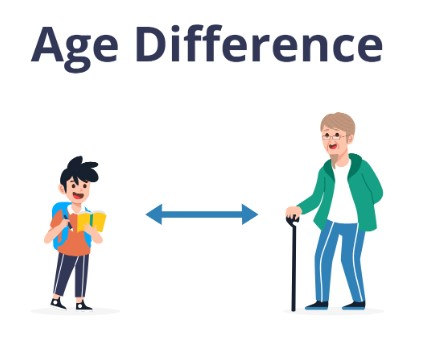# Age Difference Calculator

Enter the age of person 1 and person 2 to calculate difference in ages using age difference calculator

Give Us Feedback

## Age Difference Calculator

An age difference calculator helps to determine the difference in age between two people by their birthday. The difference will be calculated in years, months and days.## How to use this calculator?

To use this calculator, follow these simple steps

• Select Birth date of the first person from DOB of person 1 option.
• Select Birth date of the second person from DOB of person 2 option.
• Click the “Calculate” Button.

## How does this calculator work?

Our calculator first converts the total age into days. Using following formulas

• Convert years to days by multiplying it with 365
• Convert months to days by multiplying months with 30

Then we find the difference in age according to days.

To convert it into months and years, divide it by 30 and 365 respectively.

## Formula for calculating age difference

The basic formula for calculating the age difference between two people is:

`Age Difference = Person A's age - Person B's age`

For example, if Person A Birth date  is Jul-10-1990  and Person B's birth date is Feb-02-2000, the age difference would be:

Age Difference: 9 years 6 months 23 days

No of Days = 3494

Elder/Younger = Person 1 is Younger than Person 2

### Math Tools